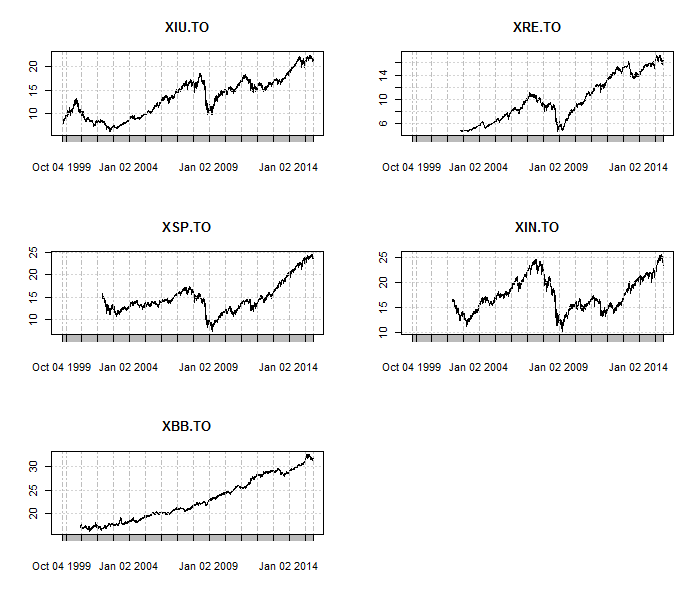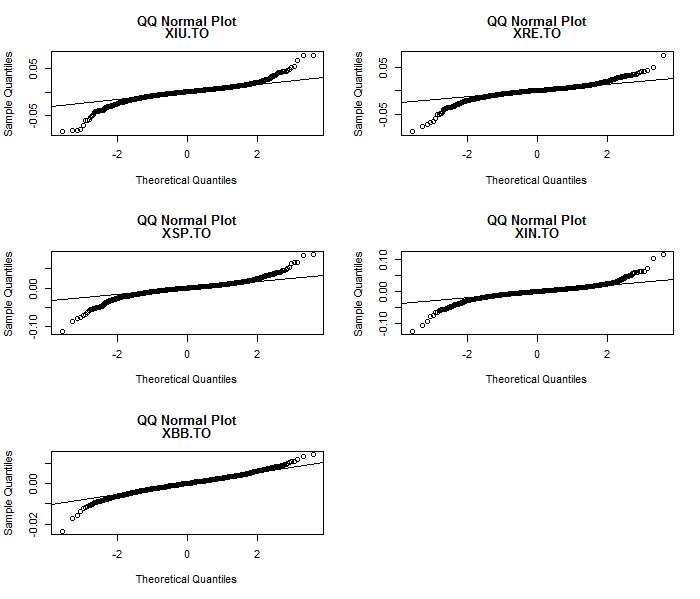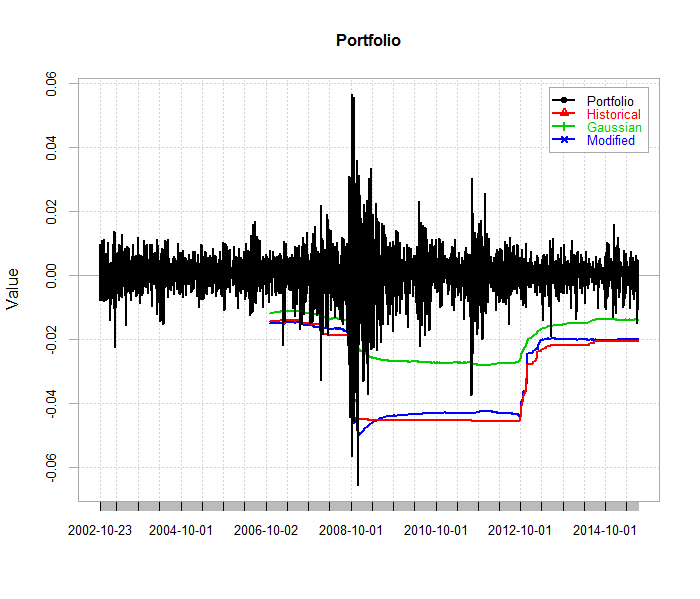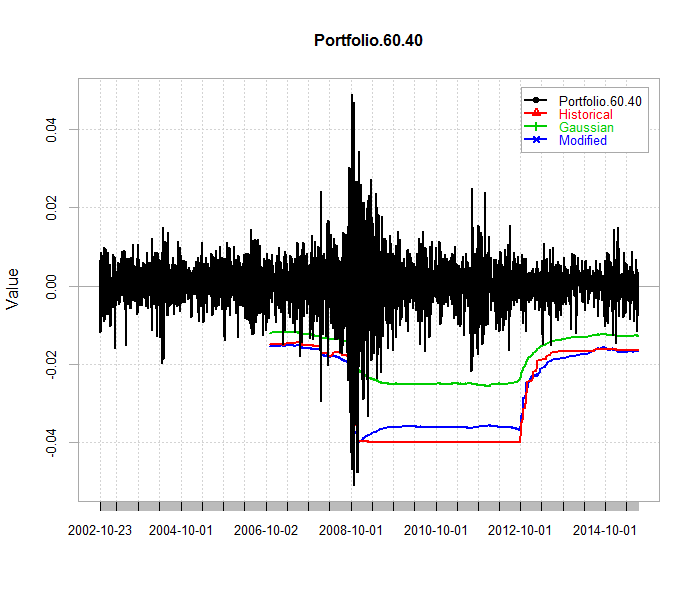Thursday, 9 July 2015

Expected Shortfall for a Canadian ETF Portfolio

In the past few weeks we have seen a lot of volatility in the financial markets. Greece is on the verge of a bailout, leaving the Euro currency, or something in between. China's stock markets, after an impressive run up over the past year have come crashing down. At times like these it is always a good idea to quantify portfolio risk. I take the position of  a Canadian investor who invests in a portfolio of broad based ETFs who wants to know their risk exposure as measured by expected shortfall (ES). ES is related to value at risk (VaR) but whereas VaR provides a measure of the minimum loss for a particular time period and coverage, ES calculates the average expected loss and is therefore closer to what an investor might expect to loose on average.

I use a portfolio of five broad asset classes: Canadian equities, Canadian REITs, US equities, Europe and Far East (EAFE) equities, and Canadian bonds. I use daily data sourced from Yahoo Finance. The ticker symbols and asset classes are as follows.

"XSP.TO", # US equities (SP500),
"XIN.TO", # EAFE equities,

Here is how each of the ETFs have performed.Except for XBB, the other ETFs experienced large drops during the 2008 - 2009 financial crisis. The Canadian bond market, however, just kept on chugging along.

Here are what the QQ plots look like for the daily continuously compounded returns.The plots indicate heavy tails for each of the asset returns. This would indicate that assuming normally distributed returns is probably not the best assumption to make when measuring risk.

Now on to calculating expected shortfall (ES). For comparison purposes, ES is calculated three ways (Historical, Gaussian, Modified). ES is calculated using a fixed width moving window of 1000 days. At the end of the day, the current ES is shifted forward by one day to provide a forecast for the next day's shortfall. Coverage is set at 99% which means that ES is calculated as the average loss over the first percentile of the return distribution. As we have seen from the QQ plots, the Gaussian approach is likely to lead to underestimating the expected shortfall because it does not account for heavy tails. In these situations, Modified often works well because it takes into account skewness and excess kurtosis.

The first portfolio I consider is a diversified portfolio with the following portfolio weights.

XIU 30%
XRE 10%
XSP 20%
XIN 10%
XBB 30%Expected shortfall calculated from the Historical or Modified versions is very similar. In comparison, ES calculated from the Gaussian approach results in more violations.

Here are what the recent daily ES values look like.

```           Historical Gaussian Modified
2015-06-30   -0.02057 -0.01398 -0.02016
2015-07-02   -0.02057 -0.01398 -0.02016
2015-07-03   -0.02057 -0.01398 -0.02016
2015-07-06   -0.02057 -0.01397 -0.02016
2015-07-07   -0.02057 -0.01395 -0.02018
2015-07-08   -0.02057 -0.01395 -0.02017```

The ES modified value for July 8, 2015 indicates that on that day there was a 1% chance of loosing an average of 2.017% of the portfolio. In other words, the average daily loss on a \$100,000 portfolio is \$2017.

For comparison purposes, I also estimate expected shortfall for a traditional 60/40 portfolio (60% XIU and 40% XBB).As in the previous case, the Historical and Modified ES values are similar while the Gaussian ES values lead to more rejections. The most recent value for the Modified ES indicates an average portfolio loss of 1.676% at 99% coverage. The average loss on a \$100,000 portfolio is \$1676. Notice that the ES values from the 60/40 portfolio are smaller in absolute value than those from the 5 asset portfolio. Interestingly, the Canadian 60/40 portfolio provides less risk than the diversified five asset portfolio.

```           Historical Gaussian Modified
2015-06-30    -0.0164 -0.01278 -0.01682
2015-07-02    -0.0164 -0.01278 -0.01681
2015-07-03    -0.0164 -0.01278 -0.01680
2015-07-06    -0.0164 -0.01278 -0.01680
2015-07-07    -0.0164 -0.01277 -0.01676
2015-07-08    -0.0164 -0.01277 -0.01676```

While the most recent volatility in the financial markets has been a concern, there has been no breaching of the Historical or Modified expected shortfall values in either the five asset portfolio or the 60/40 portfolio.

The R code is provided below. It takes about 12 minutes to complete one rolling ES calculation.

#########################################################
#  Economic forecasting and analysis
#  ES for a Canadian portfolio
#  July 2015
##########################################################

rm(list=ls())
library(fpp)
library(quantmod)

symbols = c(
"XSP.TO", # US equities (SP500),
"XIN.TO", # EAFE equities,
)

getSymbols(symbols, from="1970-01-01")
m = length(symbols)
for (i in 2:m) Y = cbind(Y, Ad(get(symbols[i])))
tail(Y)

par(mfrow = c(3, 2))
for (i in 1:m) plot(Y[, i],  main = symbols[i])
par(mfrow = c(1, 1))

## returns
y.ret <-  (na.omit(1 * diff(log(Y)) ))

par(mfrow = c(3, 2))
for (i in 1:m) {qqnorm(y.ret[, i],  main = c("QQ Normal Plot",symbols[i]))
qqline(y.ret[, i])}
par(mfrow = c(1, 1))

tail(y.ret)
weights <- c(0.3, 0.1, 0.2, 0.1, 0.3)
##########################################################

w.e = 1000
p.v = 0.99
port.ret = weights*y.ret[,1] + weights*y.ret[,2] + weights*y.ret[,3]+ weights*y.ret[,4] + weights*y.ret[,5]
names(port.ret) = c("Portfolio")

## create custom function
bt_p_ES <- function(x, p, w) {
modified.ES = as.numeric  ( ES(x, p=p, method="modified", portfolio_method = "component", weights = w)\$MES)
historical.ES = as.numeric  (ES(x, p=p, method="historical", portfolio_method="component", weights = w)\$`-r_exceed/c_exceed`)
gaussian.ES = as.numeric  ( ES(x, p=p, method="gaussian",  portfolio_method = "component", weights = w)\$ES)
ans = c(historical.ES, gaussian.ES, modified.ES)
names(ans) = c("Historical", "Gaussian", "Modified")
return(ans)
}

## rolling analysis
tic <- Sys.time()
ret.es_1 <- rollapply(y.ret, width = w.e, FUN = bt_p_ES, p=p.v, w=weights, by.column = FALSE,
align = "right"  )
toc <- Sys.time()
toc - tic

View(ret.es_1)
tail(ret.es_1)

## all three together with actual portfolio returns
ES.results = lag(ret.es_1, k=1)
chart.TimeSeries(merge(port.ret, -ES.results), legend.loc="topright")
tail(-ES.results)

##########################################################
##### comparison with 60/40 portfolio
##########################################################
port.ret.b = 0.6*y.ret[,1]  + 0.4*y.ret[,5]
names(port.ret.b) = c("Portfolio 60/40")
y.ret.b = y.ret[,c(1,5)]
tail(y.ret.b)

## rolling analysis
tic <- Sys.time()
ret.es_1.b <- rollapply(y.ret.b, width = w.e, FUN = bt_p_ES, p=p.v, w=c(.6,.4), by.column = FALSE,
align = "right"  )
toc <- Sys.time()
toc - tic

View(ret.es_1.b)
tail(ret.es_1.b)

## all three together with actual portfolio returns
ES.results.b = lag(ret.es_1.b, k=1)
chart.TimeSeries(merge(port.ret.b, -ES.results.b), legend.loc="topright")
tail(-ES.results.b)

1 comment:

1.eToro is the ultimate forex broker for newbie and professional traders.